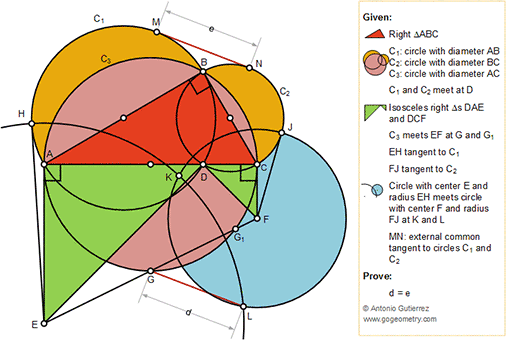## Saturday, November 17, 2018

### Geometry Problem 1401: Right Triangle with three circles on the sides, Isosceles, Diameter, Center, Tangent, Congruence

Geometry Problem. Post your solution in the comment box below.
Level: Mathematics Education, High School, Honors Geometry, College.

Details: Click on the figure below.#### 1 comment:

1.It is easy to see that
e^2=(b/2)^2 -[(a-c)/2]^2=ac/2=Area of Tr. ABC

Lets assume G' is midpoint of EF, if we draw perpendicular to AC from G', it will meet
AC at it's midpoint and its length will be (AE+CF)/2=AC/2. G' lies on circle c3, hence G' must coincide with G.
We have LG as median of Triangle ELF.
d^2=(EL^2+FL^2-2EG^2)/2
where EL=EH and FL=FJ.
Lets assume EA extended meets c1 at point Q, then AQBD is a rectangle and AQ=BD
EH^2=EA.(EA+AQ)=EA^2+EA.BD .......(1)
Similarly FJ^2=FC^2+FC.BD ........(2)
Since Tr. EDF is right Triangle and G is midpoint of EF,
2EG^2=EA^2+FC^2 .......(3)
Using eq. (1),(2) and (3) we get d^2=(EA.BD+CF.BD)/2=AC.BD/2=Area of Tr.ABC

Hence d=e